# Working with legends

## Legend functions

• Extract legend: `g_legend`
• Reposition legend onto the actual plot: `reposition_legend`
• Combine multiple plots, but use only one legend: `grid_arrange_shared_legend`

## Reposition legend onto plotting panel

`ggplot2` by default places the legend in the margin of the entire plot. This is in many instances a nice solution. If this is not desired, `theme(legend.position)` can be used to place the legend in relative measures on the entire plot:

``````library(ggplot2)
library(grid)
library(gridExtra)
dsamp <- diamonds[sample(nrow(diamonds), 1000), ]
(d <- ggplot(dsamp, aes(carat, price)) +
geom_point(aes(colour = clarity)) +
theme(legend.position = c(0.06, 0.75))
)``````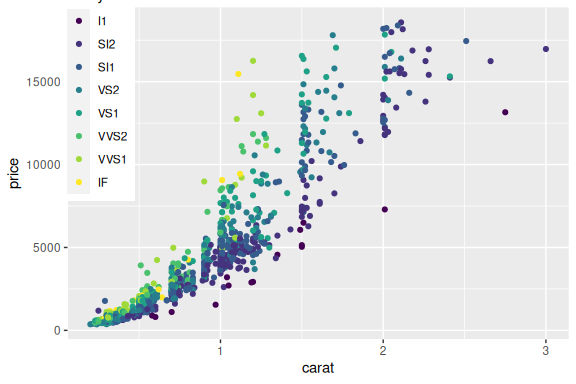Imprecise positioning of legend with `theme(legend.position)`.

This is however prone to badly positioning, if e.g. the plot is resized or font size changed: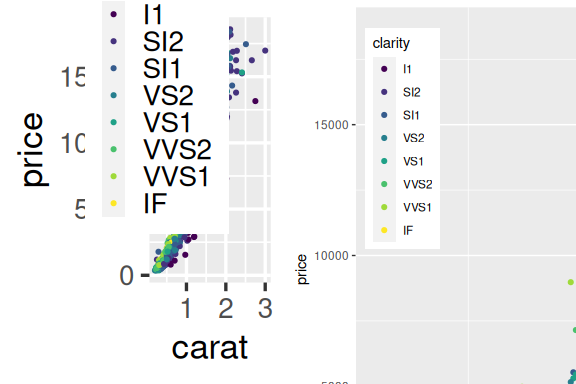Left: Base font size set to 22 pt. Right: Zoom on plot that is plotted at 150% size.

With our function, we can specify exactly how we want it in the plotting area:

``````library(lemon)
reposition_legend(d, 'top left')``````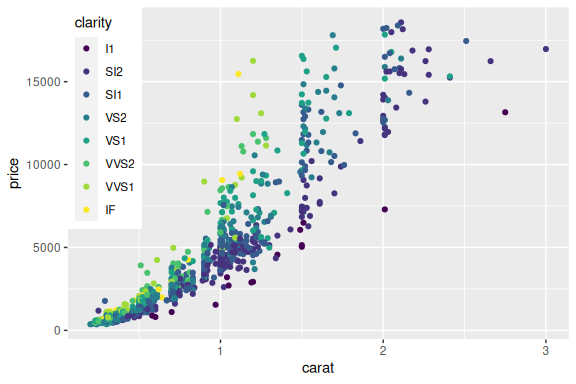Exact positioning of legend in the main panel.

And it stays there.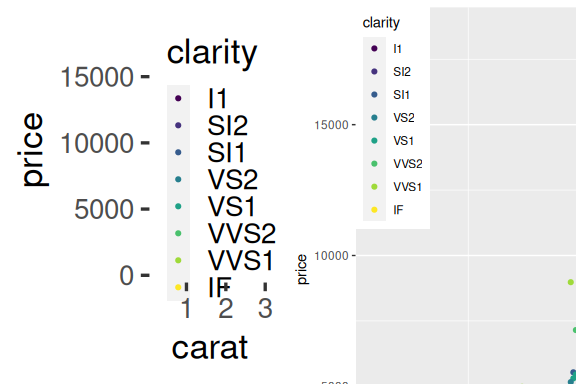Left: Base font size set to 22 pt. Right: Zoom on plot that is plotted at 150% size.

The left plot is printed in full size at the end of this document.

### Multiple legends per guide

For our final trick in this act, we reposition a legend with multiple guides. For this, use `theme(legend.box.background)` to put a background around the entire legend, not just the individual guides.

``````d2 <- d + aes(shape=cut) +
theme(legend.box.background = element_rect(fill='#fffafa'),
legend.background = element_blank())
reposition_legend(d2, 'left')``````
``````## Warning: Using shapes for an ordinal variable is not advised
## Using shapes for an ordinal variable is not advised``````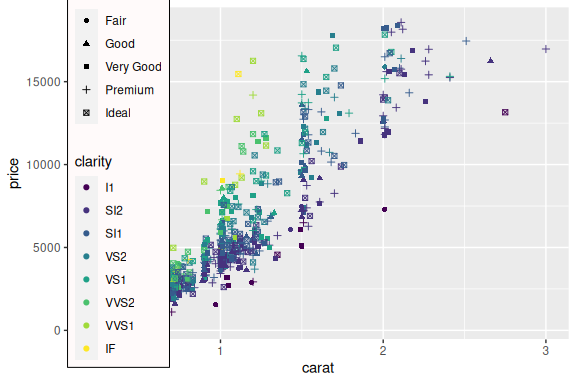Legend with multple guides on a tacky ‘snow’ background.

### Legends are placed under axis lines

The guidebox uses a solid background (subject to the chosen theme), and prior to lemon version 0.3.1, the entire legend was placed as the top most element. In the examples above, this was not an issue. With axis lines drawn, this effectively overpainted some of the axis (same applies to the panel border).

The guidebox is therefore placed under the lowest axis line, if and only if `z = Inf`. To place as top most, specify a large z-index.

``reposition_legend(d + theme_classic(), 'top left')``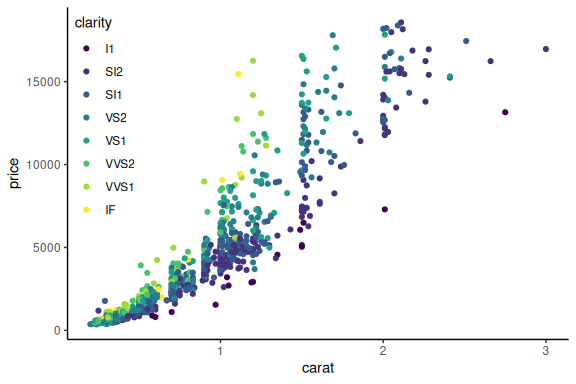Legend is drawn under axis lines.

To adjust the guidebox so it does not overpaint the panel border, use arguments `x` and `y`,

``reposition_legend(d + theme_bw(), 'top left', x=0.002, y=1-0.002)``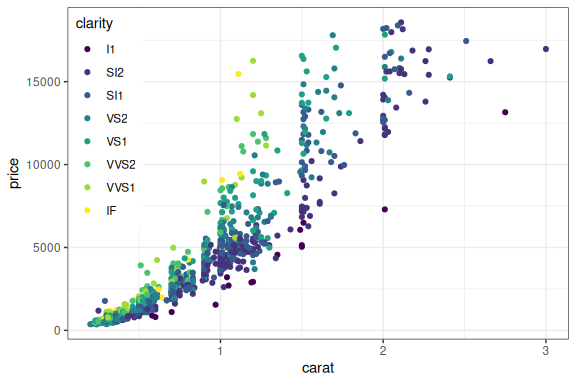Legend has to be nudged to not overpaint panel border.

… or use the argument `offset`:

``reposition_legend(d + theme_bw(), 'top left', offset=0.002)``Legend has to be nudged to not overpaint panel border, this time by `offset`.

### Warning regarding extracting legend

To our knowledge, there exists two methods for extracting the legend:

``````g1 <- function(a.gplot){
if (!gtable::is.gtable(a.gplot))
a.gplot <- ggplotGrob(a.gplot)
leg <- which(sapply(a.gplot\$grobs, function(x) x\$name) == "guide-box")
a.gplot\$grobs[[leg]]
}
g2 <- function(a.gplot){
if (!gtable::is.gtable(a.gplot))
a.gplot <- ggplotGrob(a.gplot)
gtable::gtable_filter(a.gplot, 'guide-box', fixed=TRUE)
}``````

There is very little difference between them, as the latter essentially does the same as the former. The latter however encapsulated the former in a gtable. This is even more evident with multiple guides:

``````(da <- ggplot(dsamp, aes(carat, price)) +
geom_point(aes(colour = clarity, shape=cut)) +
theme(legend.box = 'horizontal')
)``````
``## Warning: Using shapes for an ordinal variable is not advised``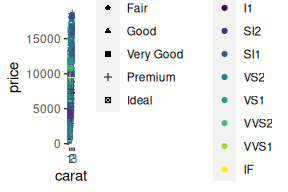Two guides in a single legend, in a grossly undersized figure.

``print(g1(da))``
``## Warning: Using shapes for an ordinal variable is not advised``
``````## TableGrob (5 x 7) "guide-box": 3 grobs
##                                     z     cells                  name
## 99_381f32e9ea4e3b1d599570c517bab0e6 1 (3-3,3-3)                guides
##                                     0 (2-4,2-6) legend.box.background
##                                               grob
## 99_381f32e9ea4e3b1d599570c517bab0e6 gtable[layout]
##                                     zeroGrob[NULL]``````
``print(g2(da))``
``## Warning: Using shapes for an ordinal variable is not advised``
``````## TableGrob (1 x 1) "layout": 1 grobs
##    z     cells      name              grob
## 1 14 (1-1,1-1) guide-box gtable[guide-box]``````

The function `reposition_legend` assumes the method given in `g1`, which is also given in `g_legend`.

### Placing the legend in facets

The above demonstration finds the panel named `panel`. This is default. If using facetting, the panels are typically named `panel-{column}-{row}`. We use `gtable_show_names` to display the names of the facetted panels.

``````d2 <- d + facet_grid(.~cut, )
gtable_show_names(d2)``````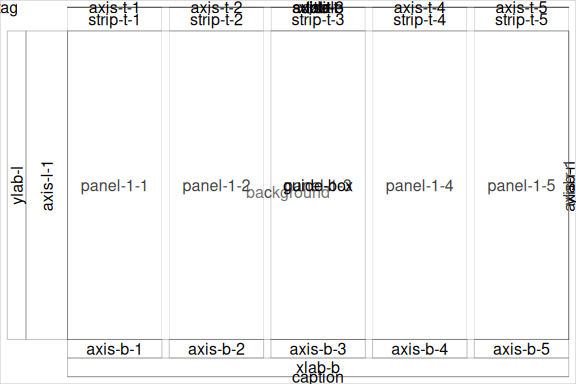Facetted panels’ names.

So to place the legend in a specific panel, give its name:

``reposition_legend(d2, 'top left', panel = 'panel-1-5')``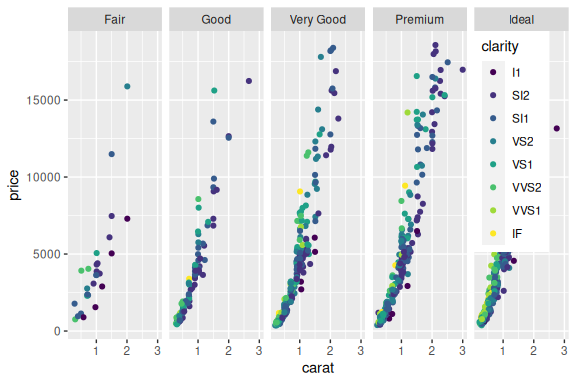Placing the legend in a facet panel.

Likewise for `facet_wrap`. Incidentally, empty panels are also named here:

``reposition_legend(d + facet_wrap(~cut, ncol=3), 'top left', panel='panel-3-2')``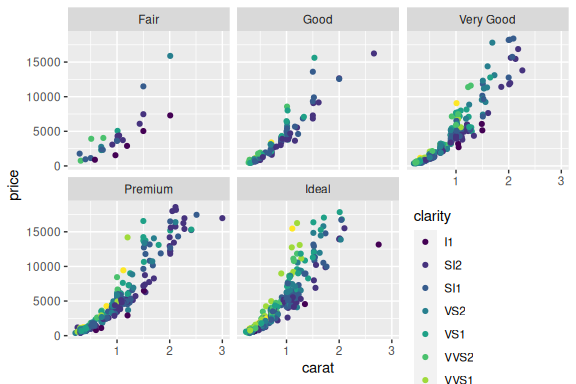Placing the legend in an empty panel when using `facet_wrap`.

Modifying the legend is done via usual routines of ggplot2:

``````d3 <- d + facet_wrap(~cut, ncol=3) + scale_color_discrete(guide=guide_legend(ncol=3))
reposition_legend(d3, 'center', panel='panel-3-2')``````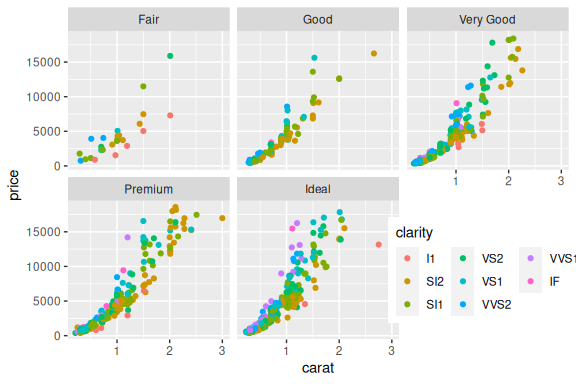The looks of the legend is modified with usual ggplot2 options.

Also supports spanning multiple panels:

``````d4 <- d + facet_wrap(~cut, ncol=4) + scale_color_discrete(guide=guide_legend(nrow=2))
reposition_legend(d4, 'center', panel=c('panel-2-2','panel-4-2'))``````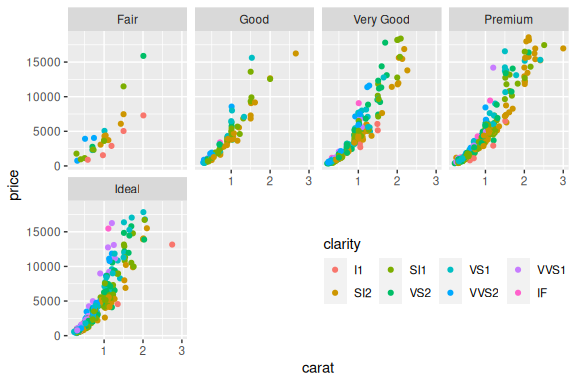Supplying `reposition_legend` with multple panel-names allows the legend to span them.

The panel names are not easy to figure, especially those from `facet_wrap`. We refer to `gtable_show_names` to get a look at where they are:

``gtable_show_names(d4)``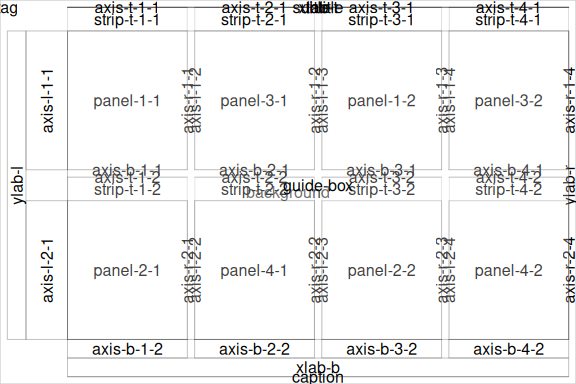Use of `gtable_show_names` to reveal the panels’ names.

## Shared legend across multiple plot

The function `grid_arrange_shared_legend` extracts the legend from its first argument, combines the plots with the legend hidden using `arrangeGrob`, and finally appends the legend to one of the sides. It even updates the plot’s theme to orientate the legend correctly.

``````dsamp <- diamonds[sample(nrow(diamonds), 1000), ]
p1 <- qplot(carat, price, data = dsamp, colour = clarity)``````
``## Warning: `qplot()` was deprecated in ggplot2 3.4.0.``
``````p2 <- qplot(cut, price, data = dsamp, colour = clarity)
p3 <- qplot(color, price, data = dsamp, colour = clarity)
p4 <- qplot(depth, price, data = dsamp, colour = clarity)
grid_arrange_shared_legend(p1, p2, p3, p4, ncol = 2, nrow = 2, position='top')``````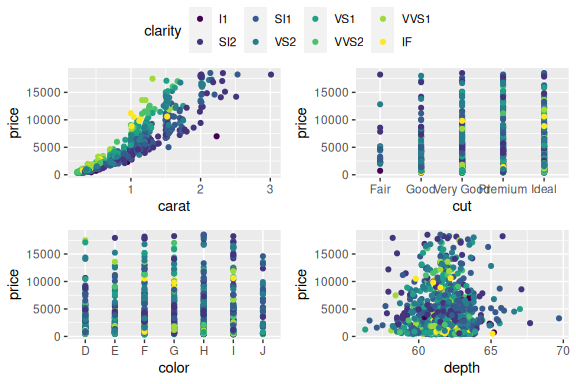``grid_arrange_shared_legend(p1, p2, p3, p4, ncol = 2, nrow = 2, position='bottom')``